Comparing different fidelity models for the impact analysis of large commercial aircrafts on a containment building a. Key Laboratory of Civil Engineering Safety and Durability of China Education Ministry, Department of Civil Engineering, Tsinghua University, Beijing, China, 100084 b. Department of Engineering Mechanics, School of Aerospace Engineering, Tsinghua University, Beijing 100084, China, 100084 Engineering Failure Analysis, 2015, 57: 254-269. DOI:10.1016/j.engfailanal.2015.08.002 Download Full Text Abstract: Three Boeing 767 finite element models with different fidelities are built using CATIA, Hypermesh and LS-DYNA in this study. The impacts of these models on a rigid wall and a containment building are simulated with LS-DYNA. The simulation results show that the time histories of the impact forces and impulses differ significantly among these models for the low-speed impact case. With the increase of the impact velocity, the time histories of impact forces and the damage of the containment building become similar. Moreover, the impact on the back side of the containment building will occur only when the high fidelity model impacts the containment building with a high velocity. The over-simplified aircraft models will underestimate the impact load and induce a different failure mode compared to the high fidelity model. The internal structures of an aircraft should be accounted for in the impact simulation. This investigation provides a reference for further studies of aircraft impacts on containment buildings. Keywords: impact; large commercial aircraft; containment building; different fidelity models; finite element simulation If you need the PDF version of this paper, please email to luxinzheng@sina.com

1 Introduction

As an emerging energy, nuclear power has been widely valued and developed around the world. Since the ˇ°September 11ˇ± terrorist attack in 2001, the safety of containment structures against aircraft impacts has drawn a lot of attention worldwide. In 1968, Riera proposed the well-known RieraˇŻs equation (Equation 1) to calculate the impact force of an aircraft on a rigid wall :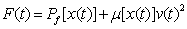(1)

in which m[x(t)] is the mass per unit length at location x, v(t) is the velocity of m[x(t)], and Pf[x(t)] is the static force required to crush the aircraft axially located at the coordinate x(t), which is controlled not only by the strength of the surface skin but also by the internal structures of the aircraft. RieraˇŻs equation provides a simplified and practical method for calculating the time-history of an aircraft impact. A full scale aircraft impact experiment was then conducted by Sugano et al. , and the reliability of RieraˇŻs equation was validated according to the experimental results.

There are two main approaches to assess the aircraft impact: (1) applying the impact load calculated by RieraˇŻs equation directly on the impacted area of the containment building to perform a time history analysis and (2) using a coupled finite element (FE) model including the aircraft and the containment building to perform the simulation of the entire impact process, i.e., the aircraft-target interaction analysis method. Previous studies have shown that the impact forces calculated by RieraˇŻs equation will be over-conservative [3-4]. Therefore, the aircraft-target interaction analysis is becoming more popular because of its higher accuracy than RieraˇŻs equation. This interaction model requires the establishment of a detailed FE model of the aircraft and the containment building.

Many studies have been conducted to simulate the impact of commercial aircraft by using the aircraft-target interaction analysis method. The FE models of the aircrafts with different fidelities are used [3-11]. For example, Wierzbicki et al.  and Karim et al.  simulated the impact between the aircraft and the World Trade Center in the ˇ°September 11ˇ± terrorist attack. However, the FE models of the aircraft used in their works are oversimplified. Even the geometry of the FE models significantly diverges from the real aircraft. Lee et al.  and Arros and Doumbalski  performed the impact simulations of a Boeing 747 on structures. Note that only the surface skin of the aircraft was considered in the FE models. Although the complex geometry and mass distribution of the aircraft were considered in their works, the internal structures of the aircraft were ignored, which may limit the accuracy of the simulation results.

In addition, some high-fidelity models are used in existing studies. The modeling of the internal structures of an aircraft for impact simulation is considered to different extents. Wilt et al. built a high-fidelity F15-E aircraft model and conducted the impact analysis . The influence of aircraft fuel (i.e., internal fuel, external tanks and the conformal fuel tanks), engines and nacelles on the impact force against the reinforced concrete (RC) wall was discussed in their model. The fuel was also modeled by Jeon et al.  and Kirkpatrick et al. . However, the beams, fuselage stringers and wing ribs, which contribute a lot to the structural stiffness, are not considered in their models [8, 9]. Siefert & Henkel  and Kostov et al.  performed the impact simulation with high-fidelity FE models including all the internal structures of the commercial aircrafts. However, the differences between such high-fidelity models and the widely used simplified models [3, 5-7] lack in-depth discussions.

According to Sadique et al.ˇŻs simulation on the impact of aircrafts of different sizes , large commercial aircrafts (e.g., Boeing 747 and Boeing 767) can induce the most significant damage on and even the global failure of the containment building. This failure mode is different from the impact of smaller airplanes (e.g., F15-E and Phantom F4), which only induces local failure. It is necessary to establish the high-fidelity FE models of large commercial aircrafts and perform the corresponding impact simulations on the containment buildings.

To compare the influence of different fidelity FE models on the impact force and the damage of containment buildings, three large commercial aircraft (i.e., Boeing 767) models with different fidelities and a containment building model are established in this study. The mass distribution, internal structures and material models of a Boeing 767 are carefully considered in these models. The impact tests of an aircraft engine are simulated to validate the feasibility of the proposed simulation method. The aircraft impact simulations are then performed, and the time histories of the impact forces and impulses are compared among different fidelity FE models. This investigation can provide a reference for the further study of the impact of aircrafts on containment buildings.

2 Numerical modeling of the aircraft

Many types of commercial aircraft, such as the Boeing 747 [3, 7, 12-13], Boeing 767  and Boeing 707 [12-16], and military aircrafts, such as the Phantom F4 [2, 17] and F15-E , were used in related impact studies. The Boeing 767-200ER is selected in this study as a typical large commercial aircraft. The reasons for selecting a Boeing 767 are the following: (1) the Boeing 767 is one of the most typical large commercial aircrafts with the passenger capacity of approximately 224; and (2) a Boeing 767 is one of the two aircrafts that impacted the World Trade Center on September 11, 2001. Many related studies have been conducted using this aircraft, and substantial data are available in the literature.

The FE model of a Boeing 767 established in this study has the identical geometric shape, surface skin and internal structures as the real aircraft. Because the FE model cannot model the equipment loads of the aircraft directly, these loads are converted to masses in the structural components at the identical position according to the mass distribution of each major component in the real aircraft (Table 1). This conversion is performed to ensure the mass distribution of the FE model conforms with the real Boeing 767 (Figure 1) [18-19].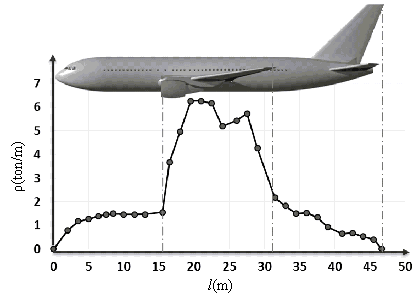Figure 1 Mass distribution of a Boeing 767

Table 1 Mass distribution of a Boeing 767

 Mass Fuselage 56.96 tons Wings 24.12 tons Engine 4.366 tons Total 113.4 tons

2.1 Modeling process

The CAD model for a Boeing 767 is first created in the three-dimensional modeling software CATIA, including the aircraft skin (Figure 2) and the internal structures. The internal details are carefully modeled following the standard data of Boeing 767, Figure 3 is provided as an example to show the details of an aircraft wing structure. The CATIA model is then converted into the general purpose preprocess program of Hypermesh to generate the FE model, in which the beams and fuselage stringers are modeled with beam elements, whereas the floors, decks, wing ribs and engines are modeled with shell elements. The shell elements and the beam elements share nodes to ensure the compatibility of displacement. Finally, the model is meshed in Hypermesh with a global mesh size of approximately 250 mm. For the components with complex geometries or force distributions (i.e. the regions where the stress distribution varies significantly, e.g., the conjunction between the engine and the wing an aircraft), the mesh size will be refined to ensure the accuracy of the simulation.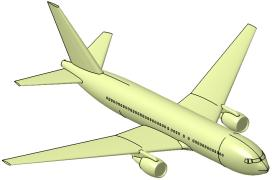(a) Surface skin of a Boeing 767-200ER (b) Geometry of a Boeing 767-200ER Figure 2 The surface skin of a Boeing 767-200ERFigure 3 The internal structures of aircraft wings

There are several FE programs that can be used for impact simulations such as LS-DYNA [4, 7], ABAQUS  and MSC.Marc [20-21]. The most widely used FE program to simulate the aircraft impact is LS-DYNA. Therefore, LS-DYNA is also selected in this study. The input file for LS-DYNA to model the aircraft is generated from Hypermesh, including approximately 50,000 Belytschko-Lin-Tsay thin shell elements  and 22,000 Hughes-Liu beam elements [23-24]. The aircraft skin and the internal structures of the FE model are shown in Figure 4. The inspection and final modification of the FE model are performed by using the pre/post-process software LS-PrePost in LS-DYNA. Because the results of the FE analysis may be affected by the element mesh, the FE mesh in Figure 4 is further subdivided to check for convergence. A series of FE meshes has been studied. Specifically, the impacts of the FE models on a rigid wall with different mesh sizes have been simulated and the time histories of the impact force compared. It has been found that the 250 mm mesh starts to converge and further refinement (i.e. 125 mm) does not lead to significant improvement to the solution. Therefore, a global mesh size of 250 mm is chosen in the simulation.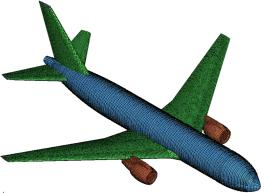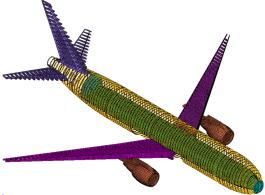(a) Surface skin (b) Internal structures Figure 4 The high-fidelity FE model of a Boeing 767

2.2 Material model

The main structural materials of a Boeing 767 are aluminum alloy and steel. The rate effect of metal cannot be ignored in the impact analysis. The most common used metal material models for impact simulations include the Plastic Kinematic model, the Piecewise Linear Plasticity model and the Johnson-Cook model, corresponding to Mat03, Mat24 and Mat15, respectively, in LS-DYNA . Existing studies related to aircraft impacts showed that the Johnson-Cook model  is the most suitable material model for simulation. This model is specially designed for large strain rate situations [7, 17, 27]. The equations of the Johnson-Cook model is shown in Equations 2 and 3 :

 (2)(3)

where e is the equivalent plastic strain,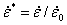is the dimensionless plastic strain rate for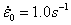, and T* is the homologous temperature. A, B, n, C and m are material constants.

The rupture strain er is given by Equation 4: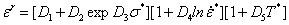(4)

in which s* is defined as sm/s, where sm and s  are the hydraulic stress and the von Mises equivalent stress, respectively. And D1 to D5 are five material constants.

All metal materials in the shell elements adopt the Johnson-Cook model (i.e., Mat15 in LS-DYNA) in this study. The typical material parameters of the Johnson-Cook model are shown in Table 2 and Table 3 according to Karim et al. . The corresponding stress-equivalent plastic strain curves are shown in Figure 5.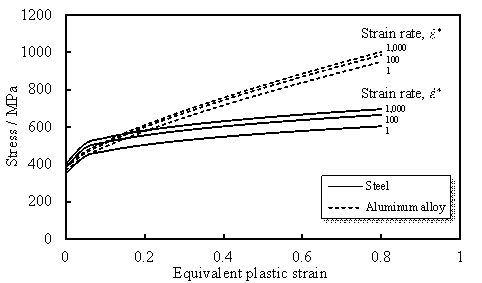Figure 5 Stress-equivalent plastic strain curves of aluminum alloy and steel

Table 2 Material properties of steel and aluminum alloy

 Density, r (t/mm3) Elastic modulus, E (MPa) Shear modulus, G (MPa) PoissonˇŻs ratio,  u Aluminum alloy 2.8´10-9 7.19´104 2.78´104 0.33 Steel 7.8´10-9 2.1´105 7.7´104 0.3

Table 3 Parameters of MAT015

 A B C m n D1 D2 D3 D4 D5 Aluminum alloy 369 684 0.0083 1.7 0.73 0.13 0.13 -1.5 0.011 0 Steel 350 275 0.022 1.0 0.36 0.05 3.44 -2.12 0.002 0.61

However, the Johnson-Cook model cannot be used for beam elements in LS-DYNA. Therefore, another widely used material model, the Plastic Kinematic model (i.e., Mat03 in LS-DYNA) , is adopted for the aluminum alloy beams in the aircraft [4, 29-30]. The stress-strain curve of the Plastic Kinematic model is bi-linear. The yield stress is defined by Equation 5: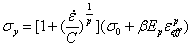(5)

where p and C are material constants,is the strain rate, Ep is the plastic hardening modulus, and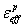is the effective plastic strain. b is a parameter to define the hardening rule of the material. The corresponding typical material parameters are shown in Table 4 according to Liu and Zheng .

Table 4 Parameters of MAT03

 Yield strength, s0 (MPa) Tangent modulus,  Et (MPa) b C p Aluminum alloy 503 5000 0 6500 4

2.3 FE models with different fidelities

In existing aircraft impact analyses, the FE models of the aircrafts with different fidelities are used. The modeling of internal structures of an aircraft is considered to different extents [4, 8-11]. In order to identify the influence of internal structures on the simulation results, three FE models with different fidelities are established.

Among these three aircraft models, Model 1 is the most detailed FE model of a Boeing 767 with the highest fidelity. All of the beam elements and shell elements that might contribute to the stiffness or strength of the aircraft are established in this model. This kind of model has been used in some of the existing literatures [10-11]. Model 2 considers all of the shell elements inside the aircraft including the wing ribs, floors and decks. However, the beam elements, such as the fuselage frames and stingers, are not modeled in Model 2. This model is similar to the FE models built by Jeon et al.  and Kirkpatrick et al . Model 3 is a surface skin shell model, in which the internal structures are ignored. This kind of model is also widely used in previous studies, too [3, 7]. Expect for the structures, these models have the identical mass distribution which is consistent with the actual aircraft (Figure 1). The comparison of these three models is shown in Figure 6.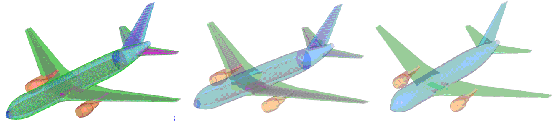(a) Model 1 (b) Model 2 (c) Model 3 Figure 6 FE models with different fidelities

In previous studies related to aircraft impacts, the targets mainly involved RC walls [2, 4, 29], rigid walls [7, 29] and containment buildings [7, 12-16]. To determine the relationship between the impact force and the fidelity of the FE model, simulations of aircrafts impacting a rigid wall and a containment building are performed in this work.

3 Impact between different aircraft models on a rigid wall

In this section, the impact forces of different aircraft models on a rigid wall are discussed. Although a rigid wall does not exist in the real world, such an idealized situation is convenient to demonstrate the influence of the aircraft models on the impact process.

From parametric discussions prior to this work, a lower impact velocity will induce a larger dispersion of impact forces among different models. The takeoff speed and the cruising speed of a Boeing 767 are approximately 77 m/s and 236 m/s, respectively. Because the height of the containment buildings is much lower than the normal flight altitude, the impact velocity of the aircraft is likely to be lower as well. Therefore, two impact velocities of 100 m/s and 150 m/s are simulated and discussed in this section. These two velocities are also widely used in previous studies [1, 3-4, 7-8, 10, 13, 16]. The time histories of the impact forces of different models are shown in Figure 7. Note that the differences of the impulses between different models and the analytical approach are in the acceptable range of numerical errors.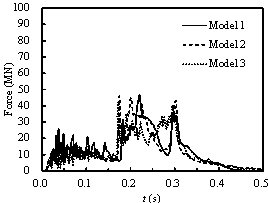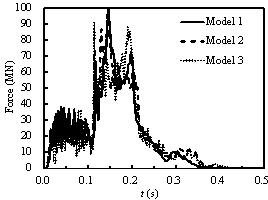(a)      v = 100 m/s (b)     v = 150 m/s Figure 7 The time histories of impact forces on a rigid wall

The results highlight differences among the impact forces of different models, and the differences are more significant under a lower impact velocity. The average impact forces of these models are calculated by Equation 6:(6)

in which tend is the total impact time. The average impact force of Model 3 is approximately 6% lower than that of Model 1 when the impact velocity equals 100 m/s. This difference reduces to 1% when the impact velocity equals 150 m/s. The reason for such differences is: according to Equation 1, the three models have the identical m[x(t)]v(t)2 during the impact process. However, Model 1 has the largest Pf[x(t)] because of the greatest stiffness among the three models. Consequently, when the impact velocity is low (i.e. 100 m/s), the influence of Pf[x(t)] of different models to the total impact force (i.e. F(t)) is more significant. When an aircraft impacts a nuclear plant, the flight attitude is low. Therefore, the aircraft likely flies at a low speed (e.g., 100 m/s). As a result, the influence of internal structures of the aircraft on the impact forces must be considered when conducting related investigations.

4 Impact between different models and a containment building

4.1 Modeling of the containment building

As mentioned above, the fidelity of aircraft models will affect the impact force. Note that the time histories of the impact forces differ when the target shape is changed . Note also that, unlike the rigid wall, the material models used in the FE model of the containment building are more complicated. Therefore, the impacts between different aircraft models and a containment building must be studied.

The nuclear power plant containment building selected in this study is a typical containment building that will be widely adopted in China. It is a double-skin steel-plates concrete containment building. The body of the containment building is a cylinder, and the dome is a hemispherical shell structure. The geometry of the containment building is shown in Figure 8. The radius of the cylinder and the hemispherical shell is 22 m. The height of the cylinder is 44 m. The wall of the containment building is 1 m thick concrete covered by two 13-mm-thick steel plates. The total height of the structure is 66 m. Shell elements are used to model the steel plates, and solid elements are used to model the concrete in the FE model. The global mesh size is 500 mm for the steel plates. For the inter concrete, the mesh size is 500 mm ˇÁ 500 mm ˇÁ 250 mm by subdividing its thickness into four layers. Similar mesh strategy is also adopted by Siefert & Henkel  and Jeon et al. . Approximately 310,000 shell elements and 620,000 solid elements are formed after meshing. The shell elements share nodes with the adjacent solid elements in the FE model of the containment building [7-8, 11-12]. In the FE model, the Johnson-Cook model (i.e., Mat15 in LS-DYNA) is used to simulate the steel, and the Concrete_Damage_Rel3 model (i.e., Mat72 in LS-DYNA) is used to simulate the concrete with a compressive strength of fc equals 48 MPa.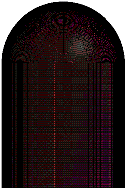Figure 8 The FE model of the nuclear power plant containment building

4.2 Validation for the material model of the containment building

The material models used in the impact simulation can be divided into two groups: (1) the metal materials and (2) the concrete material. The models for the metal materials, such as the John-Cook model and the Plastic Kinematic model, have already been widely used and validated in previous studies [4, 7, 17, 27, 29-30].

By contrast, the constitutive models of the concrete used in related studies differed from each other. For example, among the impact simulations using LS-DYNA in previous studies, different material models, such as Mat16, Mat72, Mat84 and Mat159, were used by different researchers to model the concrete [3, 29-30, 32]. In this study, the concrete of the containment building is modeled by the Concrete_Damage_Rel3 material (i.e., Mat72 in LS-DYNA). To validate the rationality of the concrete material model and the corresponding material parameters, the impact tests on a GE J79 engine conducted by Muto et al.  are simulated in this work. Although Muto et al. conducted the impact tests of different scales [33-34], only their scaled model tests considered different impact velocities (100 m/s, 150 m/s and 215 m/s), which are similar to the impact velocities of the aircraft in the subsequent simulations of this work. Furthermore, three different failure modes of the concrete panels were observed in the scaled tests. Therefore, the scaled model tests are used for the validation of the concrete material model.

A 1/7.5 ratio engine model was constructed by Muto et al. . The three tests using deformable engine models are simulated herein. The test device and the FE model are shown in Figure 9. The mass of the engine model is 3.6 kg. Eight-node solid elements and truss elements (i.e. LINK 160) are used to model the concrete and the rebars, respectively. The nodes are shared between the truss elements and the solid elements to ensure the compatibility of displacement. The material model of the rebar adopts the Plastic Kinematic model. The Belytschko-Lin-Tsay thin shell elements are used to model the engines. The material model of the engines is the Johnson-Cook model. The typical parameters of these materials are given in Table 5, and 286,000 elements are used for the entire model.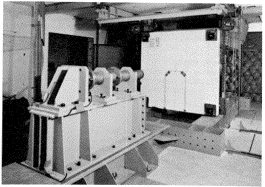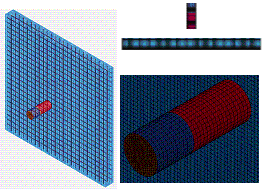(a)      Impact test device (b)     Finite element model

Figure 9 The test device and the FE model used in the engine impact tests

Table 5 Typical material parameters of the engine impact tests

 E (MPa) fc (MPa) fy (MPa) fu (MPa) Concrete / 25.5 / / Rebar 2.05ˇÁ105 / 447.2 585.1 Steel (Engine) 2.14´105 / 411.9 705.1

E is the elastic modulus of the material; fc is compressive strength of the concrete; fy and fu are the yield and ultimate strength of the rebar and the steel, respectively.

The damage of the concrete panels is simulated under the impact velocities of 100 m/s, 150 m/s and 215 m/s, respectively. The comparison between the numerical simulations and the tests is shown in Table 6. The simulation shows that when the impact velocity equals 215 m/s, the engine model perforates the target, and a 190-cm-diameter hole is left on the concrete panel (Figure 10), which is very close to the test observation. The curve of the residual velocity shown in Figure 11 is also very close to the one measured in the test (i.e., 54 m/s). When the impact velocities equal 100 and 150 m/s, no perforation occurs both in the test and the simulation. And the damage of the concrete panel from the numerical simulation is very close to the test observation (Figure 12), which further validates the concrete model of the simulation.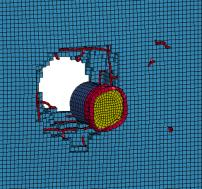Figure 10 Comparison between the simulation and the experimental result of the engine impact (v = 215 m/s)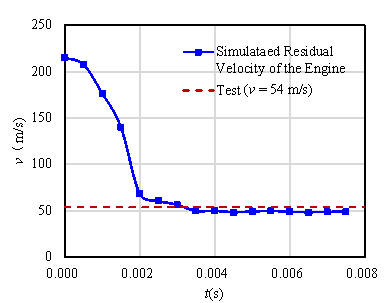Figure 11 Residual velocity of the engine after the perforation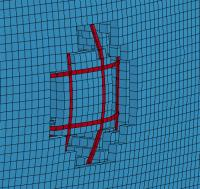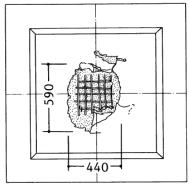Figure 12 Comparison between the simulation and the experimental result for the engine impact (v = 150 m/s)

Table 6 Results of tests and simulations of the engine impact tests

 Engine velocity Experimental result Simulation result Remark 100 m/s Penetration Penetration / 150 m/s Scabbing Scabbing Figure 10 215 m/s Perforation Perforation Figure 8

4.3 The aircraft impact simulation

In the ˇ°September 11ˇ± terrorist attack, the speed of the Boeing 767 reached 235 m/s. Among the related studies, the impact velocities are between 100 m/s and 300 m/s . Because the height of the containment buildings is much lower than the normal flight altitude, the impact velocity of the aircraft is likely to be lower as well. Therefore, impact velocities of 100 m/s, 150 m/s, 200 m/s and 250 m/s are simulated in this study corresponding to low-speed (i.e., v = 100 m/s), mid-speed (i.e., v = 150 m/s and 200 m/s) and high-speed (i.e., v = 250 m/s) situations.

4.3.1 Aircraft impact process

The entire impact process is very complicated. The impact can be roughly divided into the following four phases: (1) the fuselage impact, (2) the engines impact, (3) the wings impact and (4) the tail impact. The impact of Model 1 on the containment building with an impact velocity of 150 m/s is selected as a typical example to illustrate the process (Figures 13 and 14). First, the fuselage of the Boeing 767 impacts the containment building. The engines then impact the containment building at t = 0.15 s and cause the peak impact force. From t = 0.15 s to 0.25 s, the impact force decreases slightly because of the end of the impact from the engines. The impact force then increases again at t = 0.25 s because the wings impact the containment building. Finally, the impact force decreases from t = 0.3 s to 0.5 s and the entire process ends.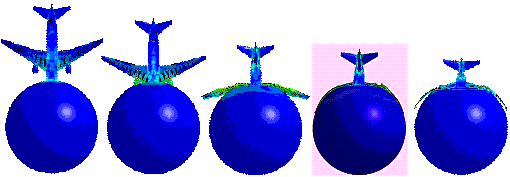(a)        t = 0.1 s (b)       t = 0.15 s (c)        t = 0.25 s (d)       t = 0.3 s (e)        t = 0.5 s

Figure 13 The simulated aircraft impact process on the containment building
(Model 1; v = 150 m/s)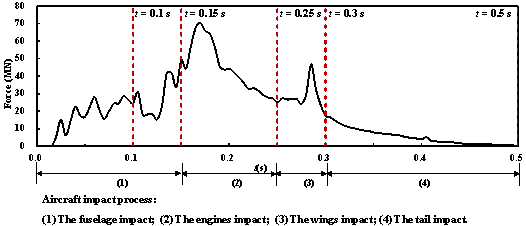Figure 14 The time history of the impact force of Model 1 on the containment building (v = 150 m/s)

4.3.2 Low-speed impact

When the impact velocity equals 100 m/s, the plastic strain of concrete is shown in Figure 15 at t = 0.45 s. It is obvious that Model 1 with the highest fidelity and internal structures causes the most severe damage to the containment building.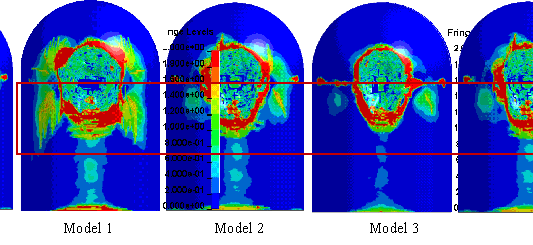Figure 15 Concrete plastic strain at t = 0.45 s (v = 100 m/s)

In Figure 15, there are two zones showing high plastic strain values. The high plastic strain values in Zone 1 are caused by the bending moment at the bottom of the containment building induced by the aircraft impact. Because Model 1 has the largest impact force, the bending moment at the bottom of the containment building induced by Model 1 is the largest among these models. The plastic strain values at the outer sides of the containment building in Zone 2 are caused by the impact of aircraft wings (Figure 16). Specifically, because the internal structures of Model 3 are not considered, the fuselage of Model 3 exhibits a significantly smaller stiffness compared to those of Model 1 and Model 2. Hence, when the aircraft impacts the containment building, the wings of Model 3 experience the largest displacements, leading to the highest plastic strain values at the outer sides for Model 3. Similarly, the beam elements of the internal structures are not modeled in Model 2 and only the shell elements are considered. The stiffness of the aircraft fuselage in Model 2 is between those of Model 1 and Model 3. As a result, the area with high plastic strain values at the outer sides of the containment building is smaller than that of Model 3 but larger than Model 1.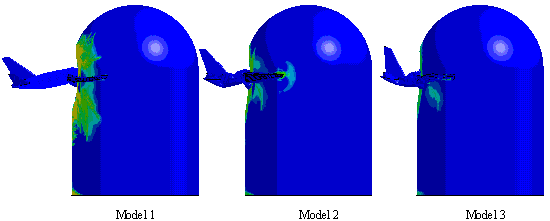Figure 16 Deformations of the FE models at t = 0.45 s (v = 100 m/s)

The impact force and impulse curves are compared among different models in Figure 17. The peak impact forces of Model 1 and Model 2 are much larger than that of Model 3, which does not consider the internal structures. Additionally, it can be found that the impulse decreases from Model 1 to Model 3. There are two main reasons for this phenomenon: (1) the scattering of the aircraft debris and (2) the erosion of the elements. Because the width of the aircraft is greater than the containment building, in the aircraft impact simulations, the debris of the aircraft will scatter during the impact process (Figure 18). Because the internal structures of Model 3 are not considered, the fuselage of Model 3 exhibits a significantly smaller stiffness compared to those of Model 1 and Model 2. Hence, when the aircraft impacts the containment building, more debris of Model 3, which will not contribute to the total impulse, will scatter, followed by Model 2 and Model 1.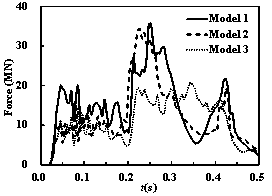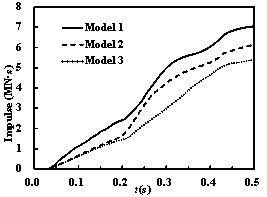Figure 17 The time histories of the impact forces and impulses on the containment building (v = 100 m/s)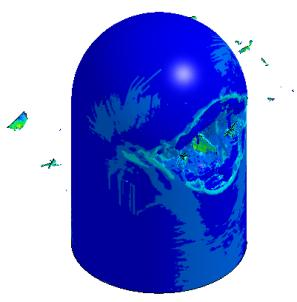Figure 18 Scattering of the aircraft debris during the impact process

In addition, the erosion of elements is considered in simulating the failure of the elements. Therefore, once the deformation of an element exceeds the corresponding threshold, it will be deleted from the FE model, which results in a continuous change of mass of the aircraft. As Model 1 has the largest stiffness, the erosion of the elements is smaller than those of the other two models. As a result, the mass participation in the impact are not identical for the models, making the impulse different for all three models.

Elements failure and scattering of the aircraft debris are also commonly found in the real aircraft impact situation  and numerical simulations, as reported in many existing literatures [7-8, 10-11]. Model 1, which considers all of the internal structures, has the largest impulse, and the impulse is almost 30% larger than that of Model 3.

4.3.3 Mid-speed impact

When the impact velocities equal 150 m/s and 200 m/s, the plastic strain distribution of the concrete is shown in Figures 19 and 20. With the increase in the impact velocity, the damage of the containment building is increasingly severe, which is consistent with the result of Lee et al. . As the impact velocity increases, the damage caused by the different models converges. The range and degree of the damage are basically identical when the velocity equals 200 m/s.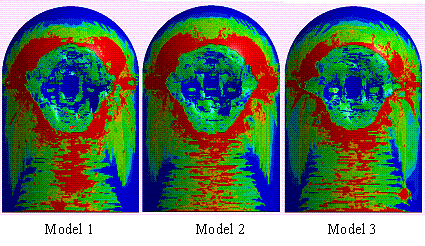Figure 19 Concrete plastic strain at t = 0.4 s (v = 150 m/s)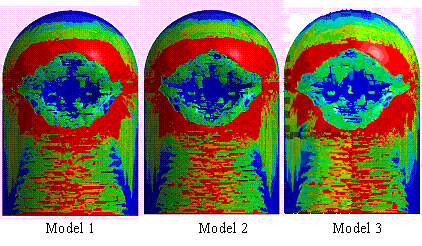Figure 20 Concrete plastic strain at t = 0.3 s (v = 200 m/s)

The time histories of the impact forces and impulses for different impact velocities are shown in Figures 21 and 22. According to the curves, the impact forces and impulses of these models increase with increasing velocities. In the mid-speed impact cases, the peak impact force of Model 1 and Model 2 are similar, whereas the peak impact force of Model 3 remains lower than that of Model 1. However, the impulse curves of these models remain different from each other. The final impulse of Model 3 is still approximately 30% lower than that of Model 1 when the impact velocity is 200 m/s.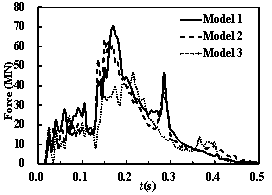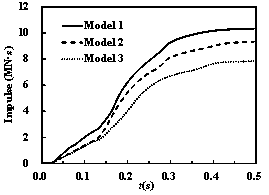Figure 21 The time histories of the impact forces and impulses on the containment building (v = 150 m/s)4.3.4 High-speed impact

When the impact velocity of the aircraft continues to increase, unlike the mid-speed impact cases, the damage of the containment building caused by different models differs from each other again. When the impact velocity equals 250 m/s, the distribution of the plastic strain of concrete at the front side of the containment building at t = 0.25 s is shown in Figure 23. The distribution of the von Mises stress of the external steel plate at the back side of the containment building at t = 0.45 s is shown in Figure 24. Although the plastic strain distribution caused by these models (Figure 23) is similar, the damage at the back side of the containment building is different among the three models. Only the fuselage of Model 1 impacts the back side, causing severe damage to the back side of the containment building. Because of the simplification of the internal structures in Model 2 and Model 3, the impact forces of the two models are smaller than that of Model 1. As a result, the critical velocities of Model 2 and Model 3 to penetrate the containment building will be larger than Model 1. Additional case studies show that the containment building will be penetrated by Model 2 and Model 3 when the initial velocities equal 300 m/s. Note that the impact force (i.e. F(t) in Equation 1) relates positively to the square of the velocity. Therefore, the impact forces at the velocity of 300 m/s are much larger than that of 250 m/s. However, due to the excessive computational cost (e.g., more than 100 gigabyte storage and 4 days for just one of the case studies), it is rather challenge to derive a critical velocity. Further studies are therefore needed to investigate the critical initial velocities of penetration for FE models with different fidelities.Figure 23 Concrete plastic strain at the front side of the containment building
at t = 0.25 s (v = 250 m/s)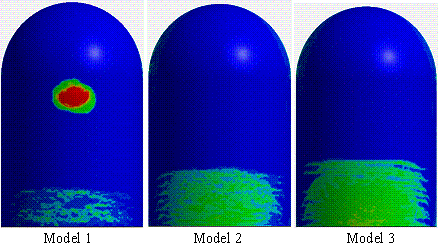Figure 24 von Mises stress of the external steel plate at the back side of the containment building at t = 0.45 s (v = 250 m/s)

When the impact velocity equals 250 m/s, the time histories of the impact forces and impulses are shown in Figure 25. The impact time of the high-speed impact cases is shorter because of the greater velocity of the aircraft. Because Model 1 entirely perforates the front side of the containment building, the impact force of Model 1 is lower than that of Model 2 and Model 3 at t = 0.2 s. However, when the fuselage of Model 1 impacts the back side of the containment building, the impact force and the impulse increase and the total impulse of Model 1 becomes larger than that of Model 2 and Model 3 again. Different failure modes of the containment building are caused by these aircraft models with different fidelities.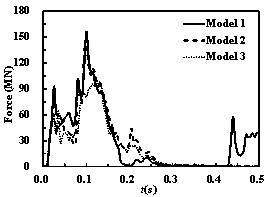Figure 25 The time histories of the impact forces and impulses on the containment building (v = 250 m/s)

4.3.5 Discussion on the simulation results

According to the simulations above, the time histories of the impact forces and impulses are closely related to the stiffness and strength of the aircraft structure. The comparison of the impact forces and impulses indicates that the impact force of Model 1 is slightly larger than that of Model 2, and both models are much larger than the most simplified Model 3. Similarly, the impulse of Model 1 is also the largest among these models during the entire impact process. The differences in the impact forces of various models generally decrease with increasing impact velocities. However, in the high-speed case, the back side of the containment building will only be impacted by Model 1 because of the perforation of the aircraft, which will induce a significantly different damage mode. The over-simplified aircraft model (similar to Model 3) used in existing studies will underestimate the impact force. Therefore for security considerations, a detailed model considering the internal structures such as Model 1 is recommended in the coupling simulation of aircraft impacts.

5 Conclusions

Three Boeing 767 FE models with different fidelities and a FE model of a containment building are established in this study. The engine impact tests are simulated to validate the concrete model. The impacts of the aircraft models with different fidelities on a rigid wall and a containment building are simulated. The following conclusions can be drawn from the simulation:

(1) In the impact simulation with the rigid wall, the influence of the modeling fidelity on the impact force is more significant under lower impact velocities. When an aircraft impacts the nuclear power plant, the low flight attitude will likely cause an aircraft to fly at a low speed. As a result, modeling the internal structures of the aircraft is necessary when conducting related investigations.

(2) In the impact simulation with the containment building, the impulse of the most detailed model (i.e., Model 1) is significantly larger than the model that only considers the surface skin (i.e., Model 3). In the low-speed impact cases, the damage caused by the high-fidelity model is significantly more severe than the other models, and the peak impact force is much larger as well. As the impact velocity increases, the damage caused by these models converges. Moreover, the impact on the back side of the containment building will occur only when Model 1 impacts the containment building with a high velocity (v = 250 m/s).

As a result, the over-simplified aircraft models used in previous studies will underestimate the impact forces. A detailed model considering the internal structures is recommended in the coupling simulation of aircraft impacts.

Acknowledgement

The authors are grateful for the financial support received from the National Science and Technology Major Project (No. 2011ZX06002-10) and National Natural Science Foundation of China (No. 51222804).

References

   Riera JD. On the stress analysis of structures subjected to aircraft impact forces. Nucl Eng Des 1968; 8(4): 415-426.

   Sugano T, Tsubota H, Kasai Y, Koshika N, Orui S, von Riesemann WA, Bickel DC, Parks MB. Full-scale aircraft impact test for evaluation of impact force. Nucl Eng Des 1993; 140(3): 373-385.

   Arros J, Doumbalski N. Analysis of aircraft impact to concrete structures. Nuclear Nucl Eng Des 2007; 237(12): 1241-1249.

   Wilt T, Chowdhury A, Cox PA. Response of reinforced concrete structures to aircraft crash impact. U.S. Nuclear Regulatory Commission, 2011.

   Wierzbicki T, Teng X. How the airplane wing cut through the exterior columns of the World Trade Center. Int J Impact Eng 2003; 28(6): 601-625.

   Karim MR, Fatt MS. Impact of the Boeing 767 aircraft into the World Trade Center. J Eng Mech 2005; 131(10): 1066-1072.

   Lee K, Han SE, Hong JW. Analysis of impact of large commercial aircraft on a prestressed containment building. Nucl Eng Des 2013; 265: 431-449.

   Jeon SJ, Jin BM, Kim YJ. Assessement of the fire resistance of a nuclear power plant subjected to a large commercial aircraft crash. Nucl Eng Des 2012; 247: 11-22.

   Kirkpatrick SW, Bocchieri RT, MacNeill RA, Peterson BD, Sadek F. Modeling methodologies for assessment of aircraft impact damage to the World Trade Center towers. Transactions of 9th International LS-DYNA Users Conference, 2006, Detroit.

 Kostov M, Henkel FO, Andonov A. Safety assessment of A92 reactor building for large commercial aircraft crash. Nucl Eng Des 2014; 269: 262-267.

 Siefert A, Henkel FO. Nonlinear analysis of commercial aircraft impact on a reactor building - Comparison between integral and decoupled crash simulation. Nucl Eng Des 2014; 269: 130-135.

 Sadique MR, Iqbal MA, Bhargava P. Nuclear containment structure subjected to commercial and fighter aircraft crash. Nucl Eng Des 2013; 260: 30-46.

 Frano RL, Forasassi G. Preliminary evaluation of aircraft impact on a near term nuclear power plant. Nucl Eng Des 2011; 241(12): 5245-5250.

 Abbas H, Paul DK, Godbole PN, Nayak GC. Aircraft crash upon outer containment of nuclear power plant. Nucl Eng Des 1996; 160(1): 13-50.

 Kukreja M. Damage evaluation of 500MWe Indian Pressurized Heavy Water Reactor nuclear containment for aircraft impact. Nucl Eng Des 2005; 235(17): 1807-1817.

 Iqbal MA, Rai S, Sadique MR, Bhargava P. Numerical simulation of aircraft crash on nuclear containment structure. Nucl Eng Des 2012; 243: 321¨C335.

 Itoh M, Katayama M, Rainsberger R. Computer simulation of an F-4 Phantom crashing into a reinforced concrete wall. Computational Ballistics II 2005; 40: 207¨C217.

 Editorial committee of aircraft design manual. Aircraft design manual. Beijing: National Defense Industry Press, 2000. (in Chinese)

 Boeing Website. http://www.Boeing.com. Accessed 2013.

 Belytschko T, Lin J, Tsay CS. Explicit algorithms for nonlinear dynamics of shells. Comput Method Appl M 1984; 42(2): 225-251.

 Hughes TJR, Liu WK. Nonlinear finite element analysis of shells: Part I. three-dimensional shells. Comput Method Appl M 1981; 27(2): 167-181.

 Hughes TJR, Liu WK. Nonlinear finite element analysis of shells: Part II. two-dimensional shells. Comput Method Appl M 1981; 26(3): 331-362.

 Jiang H, Chorzepa MG. Aircraft impact analysis of nuclear safety-related concrete structures: A review. Eng Fail Anal 2014; 46: 118-133.

 Johnson GR, Cook WH. Fracture characteristics of three metals subjected to various strains, strain rates, temperatures and pressures. Eng Fract Mech 1985; 21(1): 31-48.

 Katayama M, Itoh M, Tamura S, Beppu M, Ohno T. Numerical analysis method for the RC and geological structures subjected to extreme loading by energetic materials. Int J Impact Eng 2007; 34(9): 1546-1561.

 Krieg RD, Key SW. Implementation of a time dependent plasticity theory into structural computer programs. Constitutive Equations in Viscoplasticity: Computational and Engineering Aspects 1976; 20: 125-137.

 Cox PA, Mathis J, Wilt T, Chowdhury A, Ghosh A. Assessment of structural robustness against aircraft impact at the potential repository at Yucca Mountain-progress report II, 2006.

 Namba K, Shirai K, Saegusa T. Structural evaluation of spent nuclear fuel storage facilities under aircraft crash impact-numerical study on evaluation of sealing performance of metal cask subjected to impact force. CRIEPI Research Report N07040, 2008. (in Japanese)

 Liu JB, Zheng WK. Impact load analysis on a nuclear power plant impacted by a large commercial aircraft. Journal of Vibration and Shock 2014; 33(6): 97-101. (in Chinese)

 Seo DW, Noh HC. Aircraft impact analysis of steel fiber reinforced containment building. Journal of the Computational Structural Engineering Institute of Korea 2013; 26(2): 157-164. (in Korean)

 Muto K, Tachikawa H, Sugano T, Tsubota H, Kobayashi H, Kasai Y, Koshika N, Tsujimoto T. Experiment studies on local damage of reinforced concrete structures by the impact deformable missiles - Part 1: Outline of test program and small-scale tests. Transactions of 10th International Conference on Structural Mechanics in Reactor Technology, 1989, August 14-19, California.

 Muto K, Tachikawa H, Sugano T, Tsubota H, Nagamatsu N, Koshika N, Okano M, Suzuki K, Ohrui S. Experiment studies on local damage of reinforced concrete structures by the impact deformable missiles - part 3: Full-scale tests. Transactions of 10th International Conference on Structural Mechanics in Reactor Technology, 1989, August 14-19, California.

List of Tables and Figures:

Table 1          Mass distribution of a Boeing 767

Table 2          Material properties of steel and aluminum alloy

Table 3          Parameters of MAT015

Table 4          Parameters of MAT03

Table 5          Typical material parameters of the engine impact tests

Table 6          Results of tests and simulations of the engine impact tests

Figure 1              Mass distribution of a Boeing 767

Figure 2              The surface skin of a Boeing 767-200ER

Figure 3              The internal structures of aircraft wings

Figure 4              The high-fidelity FE model of a Boeing 767

Figure 5              Stress-equivalent plastic strain curves of aluminum alloy and steel

Figure 6              FE models with different fidelities

Figure 7              The time histories of impact forces on a rigid wall

Figure 8              The FE model of the nuclear power plant containment building

Figure 9              The test device and the FE model used in the engine impact tests

Figure 10          Comparison between the simulation and the experimental result of the engine impact (v = 215 m/s)

Figure 11          Residual velocity of the engine after the perforation

Figure 12          Comparison between the simulation and the experimental result for the engine impact (v = 150 m/s)

Figure 13          The simulated aircraft impact process on the containment building (Model 1; v = 150 m/s)

Figure 14          The time history of the impact force of Model 1 on the containment building (v = 150 m/s)

Figure 15          Concrete plastic strain at t = 0.45 s (v = 100 m/s)

Figure 16          Deformations of the FE models at t = 0.45 s (v = 100 m/s)

Figure 17          The time histories of the impact forces and impulses on the containment building (v = 100 m/s)

Figure 18          Scattering of the aircraft debris during the impact process

Figure 19          Concrete plastic strain at t = 0.4 s (v = 150 m/s)

Figure 20          Concrete plastic strain at t = 0.3 s (v = 200 m/s)

Figure 21          The time histories of the impact forces and impulses on the containment building (v = 150 m/s)

Figure 22          The time histories of the impact forces and impulses on the containment building (v = 200 m/s)

Figure 23          Concrete plastic strain at the front side of the containment building at t = 0.25 s (v = 250 m/s)

Figure 24          von Mises stress of the external steel plate at the back side of the containment building at t = 0.45 s (v = 250 m/s)

Figure 25          The time histories of the impact forces and impulses on the containment building (v = 250 m/s)